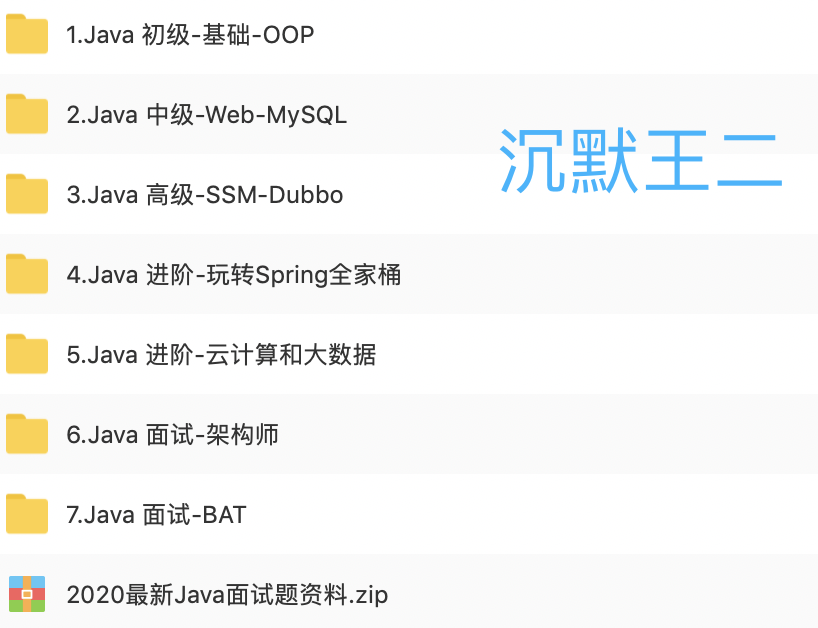01、ArrayList 是如何实现的？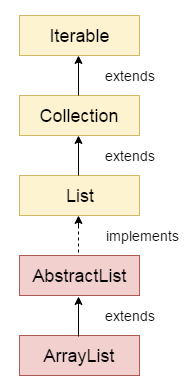ArrayList 实现了 List 接口，继承了 AbstractList 抽象类，底层是基于数组实现的，并且实现了动态扩容。

public class ArrayList<E> extends AbstractList<E>
implements List<E>, RandomAccess, Cloneable, java.io.Serializable
{
private static final int DEFAULT_CAPACITY = 10;
transient Object[] elementData;
private int size;
}

ArrayList 还实现了 RandomAccess 接口，这是一个标记接口：

public interface RandomAccess {
}

public E get(int index) {
Objects.checkIndex(index, size);
return elementData(index);
}
E elementData(int index) {
return (E) elementData[index];
}

ArrayList 还实现了 Cloneable 接口，这表明 ArrayList 是支持拷贝的。ArrayList 内部的确也重写了 Object 类的 clone() 方法。

public Object clone() {
try {
ArrayList<?> v = (ArrayList<?>) super.clone();
v.elementData = Arrays.copyOf(elementData, size);
v.modCount = 0;
return v;
} catch (CloneNotSupportedException e) {
// this shouldn't happen, since we are Cloneable
throw new InternalError(e);
}
}

ArrayList 还实现了 Serializable 接口，同样是一个标记接口：

public interface Serializable {
}

ArrayList 不想像数组这样活着，它想能屈能伸，所以它实现了动态扩容。一旦在添加元素的时候，发现容量用满了 s == elementData.length，就按照原来数组的 1.5 倍（oldCapacity >> 1）进行扩容。扩容之后，再将原有的数组复制到新分配的内存地址上 Arrays.copyOf(elementData, newCapacity)

private void add(E e, Object[] elementData, int s) {
if (s == elementData.length)
elementData = grow();
elementData[s] = e;
size = s + 1;
}

private Object[] grow() {
return grow(size + 1);
}

private Object[] grow(int minCapacity) {
int oldCapacity = elementData.length;
if (oldCapacity > 0 || elementData != DEFAULTCAPACITY_EMPTY_ELEMENTDATA) {
int newCapacity = ArraysSupport.newLength(oldCapacity,
minCapacity - oldCapacity, /* minimum growth */
oldCapacity >> 1           /* preferred growth */);
return elementData = Arrays.copyOf(elementData, newCapacity);
} else {
return elementData = new Object[Math.max(DEFAULT_CAPACITY, minCapacity)];
}
}

private void writeObject(java.io.ObjectOutputStream s)
throws java.io.IOException {
// Write out element count, and any hidden stuff
int expectedModCount = modCount;
s.defaultWriteObject();

// Write out size as capacity for behavioral compatibility with clone()
s.writeInt(size);

// Write out all elements in the proper order.
for (int i=0; i<size; i++) {
s.writeObject(elementData[i]);
}

if (modCount != expectedModCount) {
throw new ConcurrentModificationException();
}
}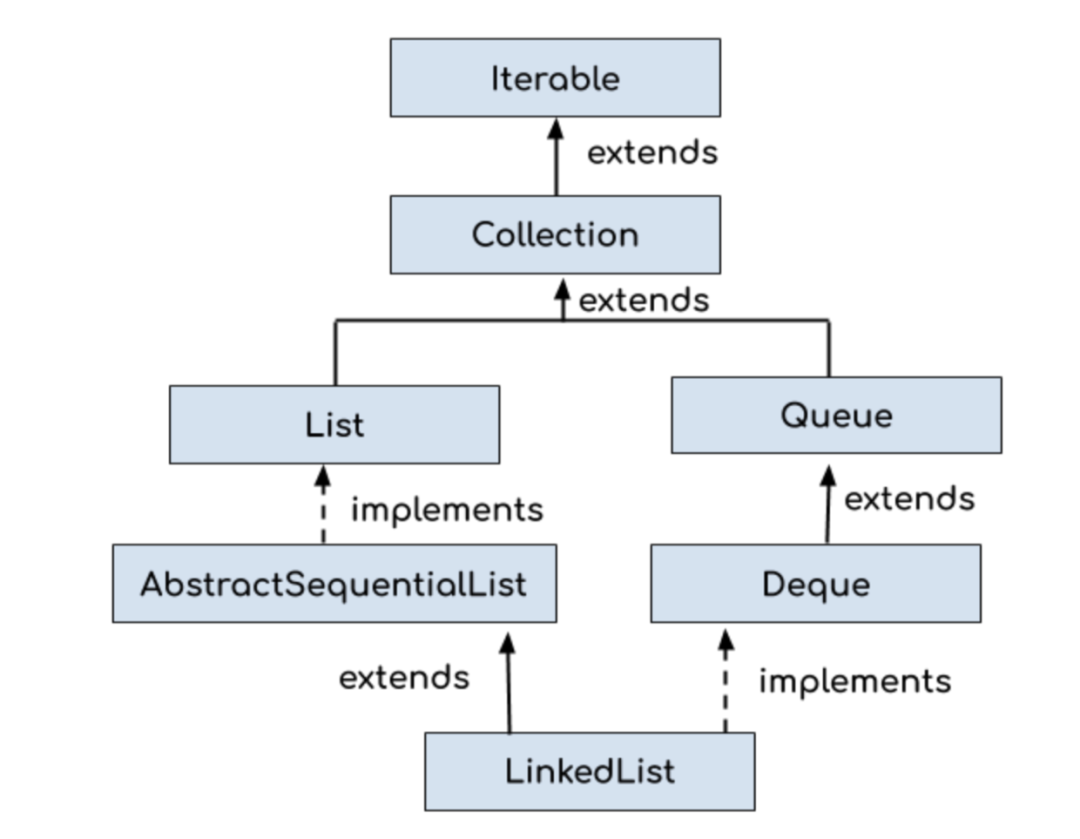extends AbstractSequentialList<E>
implements List<E>, Deque<E>, Cloneable, java.io.Serializable
{
transient int size = 0;
transient Node<E> first;
transient Node<E> last;
}

LinkedList 内部定义了一个 Node 节点，它包含 3 个部分：元素内容 item，前引用 prev 和后引用 next。代码如下所示：

private static class Node<E> {
E item;

this.item = element;
this.next = next;
this.prev = prev;
}
}

private void writeObject(java.io.ObjectOutputStream s)
throws java.io.IOException {
// Write out any hidden serialization magic
s.defaultWriteObject();

// Write out size
s.writeInt(size);

// Write out all elements in the proper order.
for (LinkedList.Node<E> x = first; x != null; x = x.next)
s.writeObject(x.item);
}

throws java.io.IOException, ClassNotFoundException {
// Read in any hidden serialization magic

// Read in all elements in the proper order.
for (int i = 0; i < size; i++)
}

last = newNode;
if (l == null)
first = newNode;
else
l.next = newNode;
size++;
modCount++;
}

1）ArrayList

ArrayList 新增元素有两种情况，一种是直接将元素添加到数组末尾，一种是将元素插入到指定位置。

modCount++;
return true;
}

private void add(E e, Object[] elementData, int s) {
if (s == elementData.length)
elementData = grow();
elementData[s] = e;
size = s + 1;
}

public void add(int index, E element) {
modCount++;
final int s;
Object[] elementData;
if ((s = size) == (elementData = this.elementData).length)
elementData = grow();
System.arraycopy(elementData, index,
elementData, index + 1,
s - index);
elementData[index] = element;
size = s + 1;
}

return true;
}
last = newNode;
if (l == null)
first = newNode;
else
l.next = newNode;
size++;
modCount++;
}

public void add(int index, E element) {
checkPositionIndex(index);

if (index == size)
else
}
// assert isElementIndex(index);

if (index < (size >> 1)) {
for (int i = 0; i < index; i++)
x = x.next;
return x;
} else {
for (int i = size - 1; i > index; i--)
x = x.prev;
return x;
}
}
// assert succ != null;
succ.prev = newNode;
if (pred == null)
first = newNode;
else
pred.next = newNode;
size++;
modCount++;
}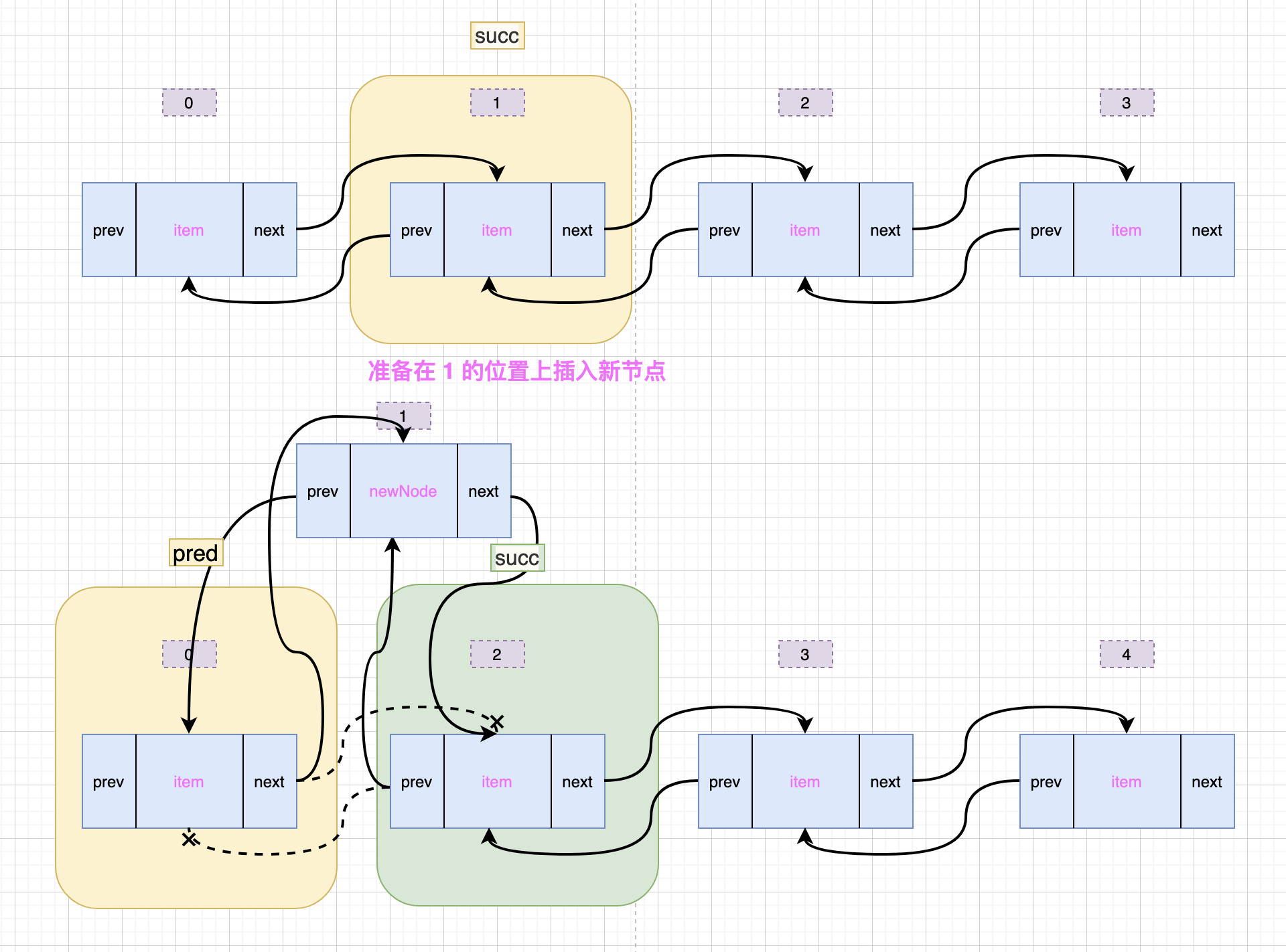public class ArrayListTest {
ArrayList<String> list = new ArrayList<String>(num);
int i = 0;

long timeStart = System.currentTimeMillis();

while (i < num) {
i++;
}
long timeEnd = System.currentTimeMillis();

System.out.println("ArrayList从集合头部位置新增元素花费的时间" + (timeEnd - timeStart));
}
}

/**
* @author 微信搜「沉默王二」，回复关键字 PDF
*/
int i = 0;
long timeStart = System.currentTimeMillis();
while (i < num) {
i++;
}
long timeEnd = System.currentTimeMillis();

}
}

num 为 10000，代码实测后的时间如下所示：

ArrayList从集合头部位置新增元素花费的时间595

public class ArrayListTest {
public static void addFromMidTest(int num) {
ArrayList<String> list = new ArrayList<String>(num);
int i = 0;

long timeStart = System.currentTimeMillis();
while (i < num) {
int temp = list.size();
i++;
}
long timeEnd = System.currentTimeMillis();

System.out.println("ArrayList从集合中间位置新增元素花费的时间" + (timeEnd - timeStart));
}
}

public static void addFromMidTest(int num) {
int i = 0;
long timeStart = System.currentTimeMillis();
while (i < num) {
int temp = list.size();
list.add(temp / 2, i + "沉默王二");
i++;
}
long timeEnd = System.currentTimeMillis();

}
}

num 为 10000，代码实测后的时间如下所示：

ArrayList从集合中间位置新增元素花费的时间1

public class ArrayListTest {
public static void addFromTailTest(int num) {
ArrayList<String> list = new ArrayList<String>(num);
int i = 0;

long timeStart = System.currentTimeMillis();

while (i < num) {
i++;
}

long timeEnd = System.currentTimeMillis();

System.out.println("ArrayList从集合尾部位置新增元素花费的时间" + (timeEnd - timeStart));
}
}

public static void addFromTailTest(int num) {
int i = 0;
long timeStart = System.currentTimeMillis();
while (i < num) {
i++;
}
long timeEnd = System.currentTimeMillis();

}
}

num 为 10000，代码实测后的时间如下所示：

ArrayList从集合尾部位置新增元素花费的时间69

1）ArrayList

ArrayList 删除元素的时候，有两种方式，一种是直接删除元素（remove(Object)），需要直先遍历数组，找到元素对应的索引；一种是按照索引删除元素（remove(int)）。

public boolean remove(Object o) {
final Object[] es = elementData;
final int size = this.size;
int i = 0;
found: {
if (o == null) {
for (; i < size; i++)
if (es[i] == null)
break found;
} else {
for (; i < size; i++)
if (o.equals(es[i]))
break found;
}
return false;
}
fastRemove(es, i);
return true;
}
public E remove(int index) {
Objects.checkIndex(index, size);
final Object[] es = elementData;

@SuppressWarnings("unchecked") E oldValue = (E) es[index];
fastRemove(es, index);

return oldValue;
}

private void fastRemove(Object[] es, int i) {
modCount++;
final int newSize;
if ((newSize = size - 1) > i)
System.arraycopy(es, i + 1, es, i, newSize - i);
es[size = newSize] = null;
}

• remove(int)，删除指定位置上的元素
public E remove(int index) {
checkElementIndex(index);
}

// assert x != null;
final E element = x.item;
final Node<E> next = x.next;
final Node<E> prev = x.prev;

if (prev == null) {
first = next;
} else {
prev.next = next;
x.prev = null;
}

if (next == null) {
last = prev;
} else {
next.prev = prev;
x.next = null;
}

x.item = null;
size--;
modCount++;
return element;
}
• remove(Object)，直接删除元素
public boolean remove(Object o) {
if (o == null) {
for (LinkedList.Node<E> x = first; x != null; x = x.next) {
if (x.item == null) {
return true;
}
}
} else {
for (LinkedList.Node<E> x = first; x != null; x = x.next) {
if (o.equals(x.item)) {
return true;
}
}
}
return false;
}

• removeFirst()，删除第一个节点
public E removeFirst() {
if (f == null)
throw new NoSuchElementException();
}
// assert f == first && f != null;
final E element = f.item;
f.item = null;
f.next = null; // help GC
first = next;
if (next == null)
last = null;
else
next.prev = null;
size--;
modCount++;
return element;
}

• removeLast()，删除最后一个节点

ArrayList从集合头部位置删除元素花费的时间380
ArrayList从集合中间位置删除元素花费的时间381
ArrayList从集合尾部位置删除元素花费的时间8

1）ArrayList

• get(int)，根据索引找元素
public E get(int index) {
Objects.checkIndex(index, size);
return elementData(index);
}

• indexOf(Object)，根据元素找索引
public int indexOf(Object o) {
return indexOfRange(o, 0, size);
}

int indexOfRange(Object o, int start, int end) {
Object[] es = elementData;
if (o == null) {
for (int i = start; i < end; i++) {
if (es[i] == null) {
return i;
}
}
} else {
for (int i = start; i < end; i++) {
if (o.equals(es[i])) {
return i;
}
}
}
return -1;
}

• get(int)，找指定位置上的元素
public E get(int index) {
checkElementIndex(index);
return node(index).item;
}

• indexOf(Object)，找元素所在的位置
public int indexOf(Object o) {
int index = 0;
if (o == null) {
for (LinkedList.Node<E> x = first; x != null; x = x.next) {
if (x.item == null)
return index;
index++;
}
} else {
for (LinkedList.Node<E> x = first; x != null; x = x.next) {
if (o.equals(x.item))
return index;
index++;
}
}
return -1;
}

// assert isElementIndex(index);

if (index < (size >> 1)) {
for (int i = 0; i < index; i++)
x = x.next;
return x;
} else {
for (int i = size - 1; i > index; i--)
x = x.prev;
return x;
}
}

for (Iterator<String> it = list.iterator(); it.hasNext();) {
it.next();
}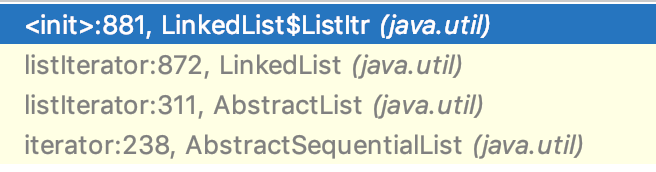public ListIterator<E> listIterator(int index) {
checkPositionIndex(index);
}

private class ListItr implements ListIterator<E> {
private int nextIndex;
private int expectedModCount = modCount;

ListItr(int index) {
// assert isPositionIndex(index);
next = (index == size) ? null : node(index);
nextIndex = index;
}

public boolean hasNext() {
return nextIndex < size;
}

public E next() {
checkForComodification();
if (!hasNext())
throw new NoSuchElementException();

lastReturned = next;
next = next.next;
nextIndex++;
return lastReturned.item;
}
}

06、总结